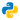# Cool New Features in Python 3.8

### Assignment expressions

Use new syntax (the “walrus operator”, :=) to assign values to variables as part of an expression ...

``````def say_hello():
print("Hello")

if (greeting := say_hello() is not None):
print(f"{greeting}, World!")

# We had been required to declare and assign value to a variable first.
greeting = say_hello()
print(f"{greeting}, World!")``````

### Positional-only parameters

Use new syntax (/) to indicate that some function parameters must be specified positionally (i.e., cannot be used as keyword arguments) ...

``````def sum(a, b , c=None, /):
result = a+b
if c is not None:
result += c
return result

>>> sum(1, 2) # valid
>>> sum(1, 2, 3) # valid

>>> sum(a=1, b=2) # invalid
>>> sum(1, 2, c=3) # invalid``````

### f-strings now support = for quick and easy debugging

f'{expr=}' expands to the text of the expression, an equal sign, then the repr of the evaluated expression ...

``````price = 6250
tax = int(6250 * 0.2)
print (f"Total price is {price + tax=} dollars.")

# We had not been able to use = in f-strings
total = price + tax
print (f"Total price is {total} dollars.")``````

### functools.lru_cache()

It can now be used as a decorator rather than as a function returning a decorator ...

``````from functools import lru_cache
@lru_cache(maxsize=None)
def fib(n):
if n < 2:
return n
return fib(n-1) + fib(n-2)

>>> [fib(n) for n in range(16)]
[0, 1, 1, 2, 3, 5, 8, 13, 21, 34, 55, 89, 144, 233, 377, 610]

>>> fib.cache_info()
CacheInfo(hits=28, misses=16, maxsize=None, currsize=16)``````

It is not necessary to write a memoization function (below) anymore.

``````def memoize(func):
cache = dict()

def memoized_func(*args):
if args in cache:
return cache[args]
result = func(*args)
cache[args] = result
return result

return memoized_func``````

Calculate the product of all the elements in the input iterable. The default start value for the product is `1`. When the iterable is empty, return the start value.
``````from math import prod